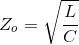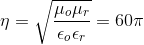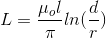# MCQs on Power Systems

##### Page 36 of 67. Go to page 1 2 3 4 5 6 7 8 9 10 11 12 13 14 15 16 17 18 19 20 21 22 23 24 25 26 27 28 29 30 31 32 33 34 35 36 37 38 39 40 41 42 43 44 45 46 47 48 49 50 51 52 53 54 55 56 57 58 59 60 61 62 63 64 65 66 67
01․ The characteristic impedance of a transmission on line is
Directly proportional to its length.
Directly proportional to square root of its length.
Inversely proportional to its length.
Independent of its length.

The characteristic impedance of a transmission line is given as,Where the inductance L and capacitance C are per unit lengths. These 'per unit length' values cancel out, and hence the characteristic impedance does not depend on the length of the transmission line.

02․ In a lossless medium the intrinsic impedance η 60π and μr = 1. The relative dielectric constant εr shall be
2.
4.
1.
8.

For free space ηo = & radiac; (μ/ε = 12 π for any lossless medium with μr= 1So ε r = 4.

03․ Which following statement are not true for line parameters R, L, G and C of transmission line
LC = μ ε and RG = σ ε.
G = I/R where G is conductance of line.
Both R and G depend on the conductivity of conductor forming the line.
All of above.

For each line LC = μ ε G/c = σ/ε.

04․ Which statement is not correct for the transmission line parameter R, L, G and C
At high frequencies at which most of communication system operate the internal inductance of the conductor is neglected.
The line parameter R,L,G and C are uniformly distributed along the entire length of the line C.
G = I/R. R is the ac resistance per unit length of conductor C.
For each line, the conductors are characterized by σ c, μ c, εo = ε c and the homog

G is not equal to I/R, where G is conductance per unit length of the conductor and is due to the dielectric medium separating the conductor.

05․ Consider a long, two wire line composed of solid round conductors. The radius of both conductors is 0.25 cm and distance between their centers is 100 cm. If this distance is doubled, then the inductance per unit length.
Halves.
Doubled.
Increase but does not doubled.
Decrease but does not halves.Where d and r distance between wires and radius respectively. Here L ∝ lnd. So, when d is doubled inductance increase but does not doubled.

06․ A synchronous generator is of 100 MVA have inertia constant of 20 MJ/MVA. Find the angular momentum in MJsec/electrical degrees
2000
5
0.22
0.4

Angular momentum M = HS/(180*f) MJsec/electrical degrees Where, H = Inertia constant S = Rating of the machine Angular momentum M = 20*100/(180*50) = 0.22 MJsec/electrical degrees

07․ Which of the following statement is/are true regarding infinite line?
It has infinite length
It has infinite voltage
It maintains the constant voltage
both 2 and 3

If all the generators are connected in parallel and maintaining constant voltage at bus bar without any internal impedance for equivalent bus bar is called infinite bus bar, i,e, it maintains the voltage constant independent of load condition.

08․ Equal area criteria is used for
relative stability of the system
absolute stability of the system
either 1 or 2
nether 1 nor 2

Equal area criterion used to transient stability analysis of power system, is consistency with transient energy function method for single machine-infinite bus system or two machines system.Equal area criteria is used more efficiently for a single generator stability analysis or single motor stability analysis and is is used to find the absolute stability of the system.

09․ Which of the following is/are the methods of improving transient stability?
Fast acting governing system
Fast acting excitation control
Dynamic breaking
all of the above

Methods of improving transient stability 1. Fast acting governing system 2. Reducing system reactance 3. Fast acting excitation control 4. Dynamic breaking 5. Single pole switching 6. Shunt compensation

10․ If a 500 MVA, 11 kV 3-phase generator at 50 Hz feeds through a transfer impedance of (0+j0.605) Ω/phase, an infinite voltage also at 11 kV, then the maximum steady state power transfer on the base of 500 MVA and 11 kV is
1 pu
0.8 pu
0.4 pu
0.5 pu

Maximum steady state power = EV/X Where, E = Sending side voltage V = Receiving side voltage X = Reactance of the line Maximum steady state power = 11*11/0.605 = 200 MW Maximum steady state power in pu = 200/MVAbase= 200/500 = 0.4 pu

<<<3435363738>>>